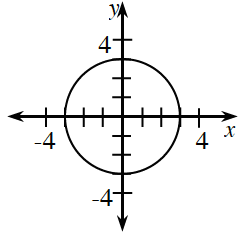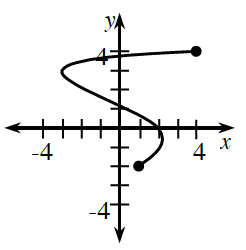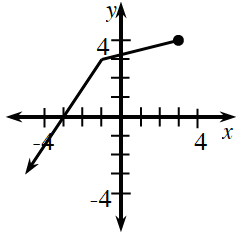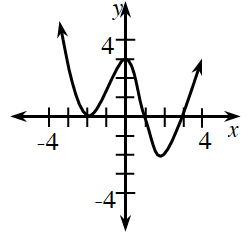### Home > CCA2 > Chapter Ch12 > Lesson 12.1.3 > Problem12-44

12-44.

State the domain and range for each graph below. Then state whether or not it is a function.

The domain is the set of all $x$-values of a relation or function.
The range is the set of all $y$-values of a relation or function.
For a relation to be a function, each $x$-value must correspond to only one $y$-value.

1.Domain: $-3 ≤ x ≤ 3$
Range: $-3 ≤ y ≤ 3$
Not a Function

1.Domain: $-3 ≤ x ≤ 4$
Find the range.
Is this a function?

1.Find the domain.
Range: $−∞ ≤ y ≤ 4$
Is this a function?

1.This is a function.
Find the domain and range.